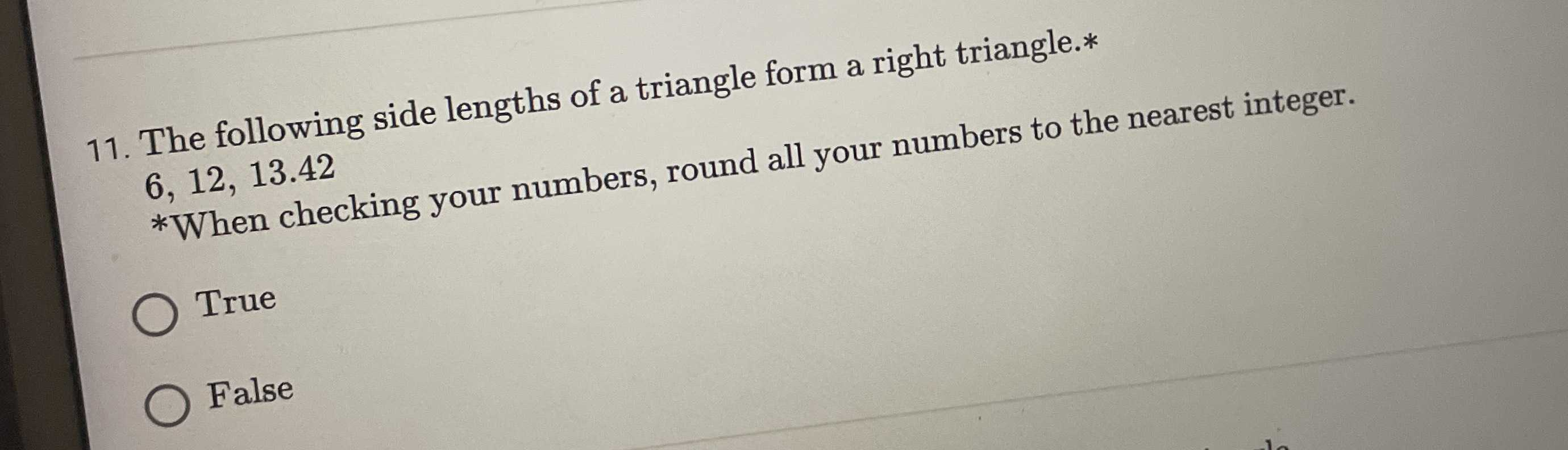### Still have math questions?

Arithmetic
Question11. The following side lengths of a triangle form a right triangle.*

$$6,12,13.42$$

*When checking your numbers, round all your numbers to the nearest integer. True False